### Home > MC2 > Chapter 7 > Lesson 7.2.1 > Problem7-68

7-68.
1.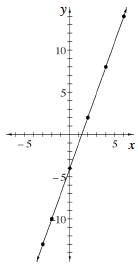Use the graph shown at right to enter the ordered pairs in the table below. Homework Help ✎

 x y ____ ____ ____ ____ ____ ____ ____ ____ ____ ____ ____ ____ ____ ____

1. Write the rule in words.

2. Explain how to use the table to predict the value of y when x is –7.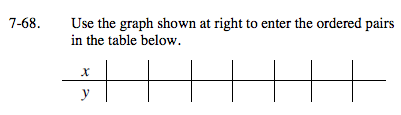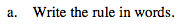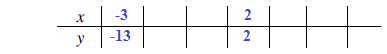Write the coordinates of the corresponding points from the graph at right. Two points have been filled in for you.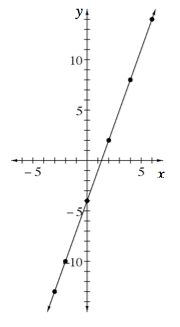Remember, try to find the rule based on the constant given by the y-value when x is 0.
Then work backwards and see how x and y are related after working in the constant.

Multiply the number by three and then subtract by four.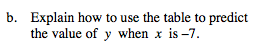If for every value of x, the y-value increases or decreases by 3, how can you compare −3 and −7?